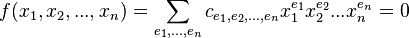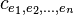# Algebraic equation

## Definition

Definition. An algebraic equation in n variables is an polynomial equation of the formwhere the coefficientsare integers, the exponents ei are nonnegative integers and the sum is finite.

### Examples.

1. The octoide: x4 - (x2 + y2) = 0

2. The unit circle: x2 + y2 - 1 = 0

3. The unit sphere: x2 + y2 + z2 - 1 = 0

Source:  Wolfram MathWord

Keywords: mathematica, algebra, algebraic equations, superior algebra

### Forum

Here you can discuss about mathematic, about algebra, geometry, trigonometry.

It is not mandatory to be logged in on this forum but it is nice to have an account. You can ask about mathematics just with your name and your email.

This maths forum is one of the easiest forums to use it.

>> Go to Math Forum